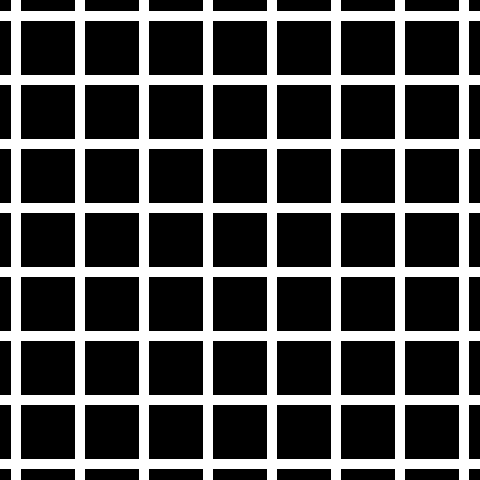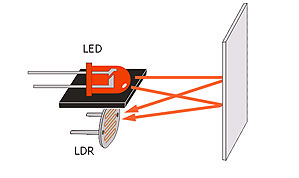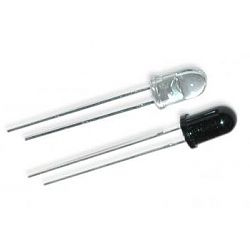# Grid Follower

#### Introduction

Grid solving robots are very useful in various robotics competitions. In this tutorial we would be dealing with how the grid follower robot works along with its algorithm. A grid follower is basically a line follower with a modified code which helps it in traversing a grid. Just as a simple line follower, a grid follower takes inputs from various sensors to detect its position on the grid.#### Robot

The robot will consisting of two parts

Sensor Part:

The sensor will be basically a light detecting sensor. We here are using the property of absorption. We all know that black surface tends to absorb all the light that falls on it. So, we place a LED(Light Emitting Diode) along with a LDR(Light Dependant Resistor) as our sensor. Suppose that our sensor is hovering upon white surface. White surface reflects more than black. Therefore, more light would be reflected on LDR and thus, its resistance would change and we can predict accordingly that the sensor will be upon white surface. When it will be hovering upon black surface, less amount of light will be reflected and so we can predict that it is over the black surface.We can use IR sensor too instead of LED-LDR sensor.Arrangement:

The Robot will be a simple line follower with additional sensors for finding nodes in the grid (Node is basically an intersection between two lines). So there will be some sensors for line following and some sensors for node detection.
–0-0-0-0-0–
|           |
|           |
|           |
0–[M]         [M]–0
|           |
————-
The above figure shows the robot along with sensor placement. The sensors are shown by “0” and the motors in the robot are shown by “[M]”. Basically the Grid follower robot will have line follower part (which includes the front sensors) and node detector (which includes the sensors parallel with motor shaft). The Grid follower has to basically follow line when it is not on a node and when a node arrives it has to decide whether to take a left or right depending upon the final position it has to reach.

Driving Part:

This part is very simple it include two motor along with a motor driver IC. Motor driver will not be explained in this tutorial. It is explained in detail in this tutorial.

#### Line Following Algorithm

The line follower is explained in another tutorial. Basically it deciding which motor to run depending upon the locations of sensors. In our code one function is made which uses the line follower algorithm.

#### Grid Following Algorithm

The Problem statement here is that given two coordinates on grid the robot has to move from one point to another. For convenience we are considering that the robot will cover the x-coordinates first and the will cover the y-coordinates. For this configuration we need to perform the following steps:
Align in proper X-direction.
Move on some nodes so that the current x-coordinate = the final x-coordinate.
Align in proper Y-direction.
Move on some nodes so that the current y-coordinate = the final y-coordinate.
With these four steps your robot will reach the final coordinate.
Example:
Your robot is at (0,0) and it need to reach (2,2). The left lower corner is (0,0) and the right upper corner in (2,2).
“[w]” means forward direction.
“[s]” means backward direction.
“[a]” means left direction.
“[d]” means right direction.
“” meas node.
|      |      |
--—-—-–
|      |      |
--—-—-–
|      |      |
--[a]-——-–
|      |      |
So currently your robot is at (0,0) and pointing in left direction.
It has to reach (2,2) first covering x-coordinates and then y-coordinates.
1] Align in proper direction.
In this case the robot must point towards right. Therefore after this step your grid along with robot will look like.
|      |      |
--—-—-–
|      |      |
--—-—-–
|      |      |
--[w]-——-–
|      |      |

|      |      |
--—-—-–
|      |      |
--—-—-–
|      |      |
--[d]-——-–
|      |      |
2] Move on some nodes so that current x-coordinate is equal to final x-coordinate.
In this case it has to move from (0,0) to (1,0) to (2,0)
|      |      |
--—-—-–
|      |      |
--—-—-–
|      |      |
--[d]-——-–
|      |      |

|      |      |
--—-—-–
|      |      |
--—-—-–
|      |      |
--—-[d]-—–
|      |      |

|      |      |
--—-—-–
|      |      |
--—-—-–
|      |      |
--—-—-[d]--
|      |      |
3] Align in proper direction.
In this case the robot must point towards top. Therefore after this step your grid along with robot will look like.
|      |      |
--—-—-–
|      |      |
--—-—-–
|      |      |
--—-—-[w]--
|      |      |
4] Move on some nodes so that current y-coordinate is equal to final y-coordinate.
In this case it has to move from (2,0) to (2,1) to (2,2)
|      |      |
--—-—-–
|      |      |
--—-—-–
|      |      |
--—-—-[w]--
|      |      |

|      |      |
--—-—-–
|      |      |
--—-—-[w]--
|      |      |
--—-—-–
|      |      |

|      |      |
--—-—-[w]--
|      |      |
--—-—-–
|      |      |
--—-—-–
|      |      |
So, we have tested that our algorithm works!!

#### The Hardware Part of the Robot

In this section the connections of sensors motor-driver and microcontroller will be explained. Before reading this section make sure that you completely understand the functioning of motor-driver IC (L293d) , LED-LDR sensor and pinouts of ATmega16.
I will be using ATmega16 for this grid follower as my microcontroller.

As we have already discussed where to place the sensors on the bot, now we will talk about its connections with the microcontroller.
The sensor will give output in the form of analog-voltage. This voltage has to be compared with some threshold voltage to decide whether the concerned sensor ie either on white or black. For this there are two methods:

1. ADC - Analog to Digital Converter:
Here we assign a digital value to certain analog voltage and then using software compare it with the threshold value. In this case the output from the sensor can be directly connected to the ADC pin of the microcontroller.
2. Comparator:
Here the analog voltage is compared using another know voltage(Vth) which can be set up using a potentiometer. Depending upon the connections the output will be either high or low. In this case the output from the comparator can be directly given as input to any I/O pin( Input / Output ) of the microcontroller.

The motors connection will be done with the motor-driver IC and the control lines of the motor driver IC are connected to the microcontroller (For more specific details refer to motor-driver tutorial)